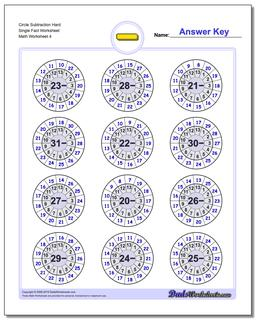# Math Worksheets: Subtraction: Subtraction: Circle Subtraction Hard Single Fact Worksheet (Fourth Worksheet)## Circle Subtraction Hard Single Fact Worksheet (Fourth Worksheet)

PropertyValue
DescriptionCircle Subtraction Hard Single Fact Worksheet: These Subtraction worksheets emphasize groups of related facts and there are variations with the facts in order to facilitate skip counting, or with random products that help facilitate fact memorization. Try the variations with all facts, or print the worksheets that focus only on specific families of multiplication facts that need more practice! (Fourth Worksheet)
Resource TypeWorksheet